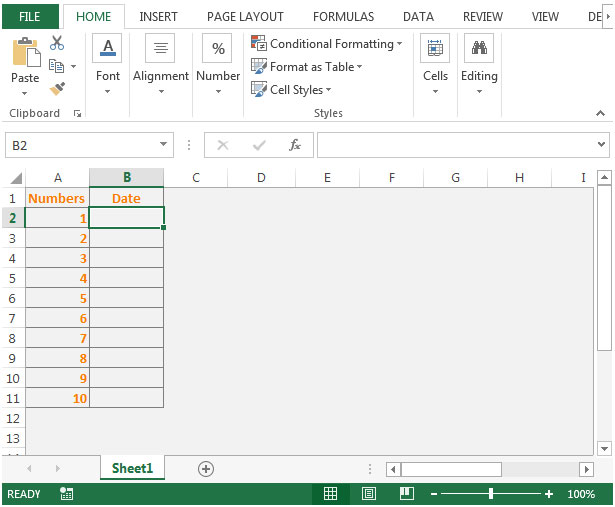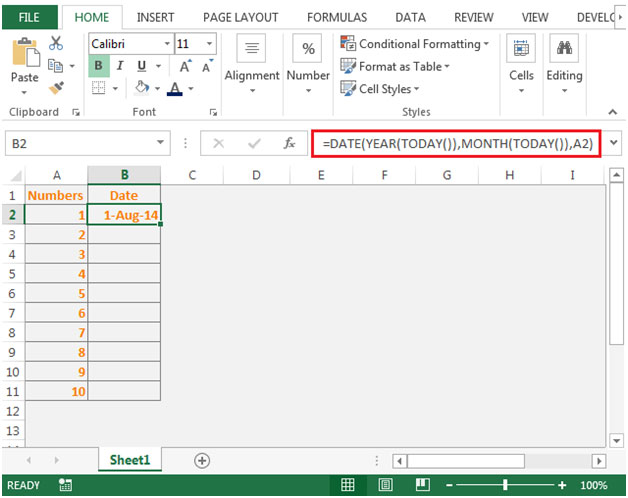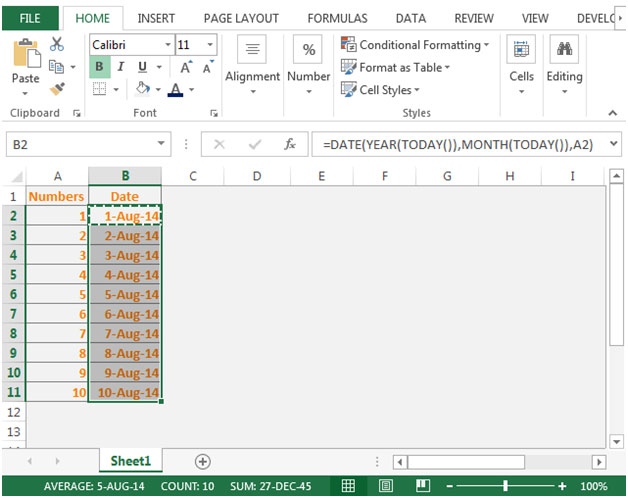# Entering Dates Quickly in Microsoft Excel 2010

In this article, we will learn how to enter dates quickly in Microsoft Excel 2010.

With dates formula we can count dates based on if the condition meets or not, we can count days between dates.

In order to enter date of a current month in a cell where excel will let you enter only a number in a cell & the formula will enter the year & month itself, the functions we can use are DATE, YEAR, MONTH, TODAY.

DATE: Returns the number that represents the date in Microsoft Excel date-time code.

Syntax: =DATE(year,month,day)

year: The year argument can include one to four digits. By default, Excel uses the 1900 date system.

month: The second argument, representing the month of the year from 1 to 12 (January to December)

day: The third argument, representing the day of the month from 1 to 31.

YEAR: Returns the year of a date, an integer in the range 1900 - 9999

Syntax: =YEAR(serial_number)

serial_number: The date of the year you want to find.

MONTH: This function returns the month (January to December as 1 to 12) of a date.

Syntax: =MONTH(serial_number)

serial_number: It refers to the date of the month that you are trying to find.

TODAY: Returns the current date formatted as a date

Syntax: =TODAY()

TODAY function does not have any argument.

Let us take an example:

• We have some numbers in column A
• In column B, the formula will calculate the date of the corresponding cells in the left column• In cell B2, the formula would be =DATE(YEAR(TODAY()),MONTH(TODAY()),A2)• The first argument of Date function is Year. YEAR(TODAY()) will return the current year i.e. 2014
• The second argument of Date function is Month. MONTH(TODAY())will return the current month i.e. August
• The third argument of Date function is taken from column A which is being Day of the month.
• By combining all of them, our formula will give the output.
• Copying the formula down to below range of cells, we will get our desired output.Terms and Conditions of use

The applications/code on this site are distributed as is and without warranties or liability. In no event shall the owner of the copyrights, or the authors of the applications/code be liable for any loss of profit, any problems or any damage resulting from the use or evaluation of the applications/code.